# Wave Walker DSP

## Brand New Book!

DSP for Beginners: Simple Explanations for Complex Numbers! The second edition includes a new chapter on complex sinusoids.

Can the Fourier Transform Magnitude Be Negative?
February 16, 2022

#### Introduction

I came across a question on DSP Stack Exchange the other day: can the Fourier Transform magnitude be negative? This is a great question! The answer is no, and let’s take a look at why.

More blogs on DSP:

#### Discrete Time Fourier Transform

The discrete-time Fourier transform (DTFT) is

(1)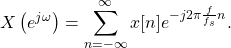The DTFT is a cross correlation of x[n] with a complex exponential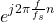. The summation over all n produces a single complex number. The DTFT is written as a real and imaginary component,

(2)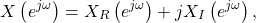where

(3)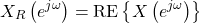and

(4)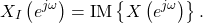#### Magnitude of Complex Number

Rather than use the complex number in (2) a simpler representation is given by

(5)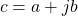where the complex number c has a real part a and an imaginary part jb. The magnitude of a complex number c can be written as

(6)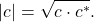The magnitude can be written in terms of a and b by substituting (5) into (6),

(7)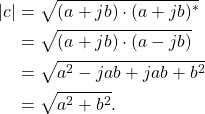The square of a real number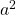and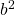cannot be negative and the square root of a positive number is always positive, therefore the magnitude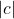cannot be negative.

#### Plotting Magnitude of Complex Number

Conceptually the magnitude of a complex number c is the length of the vector beginning at the origin 0 + 0j and ending at c. Consider a complex number

(8)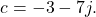Figure 1 plots the complex vector c in 2D complex space.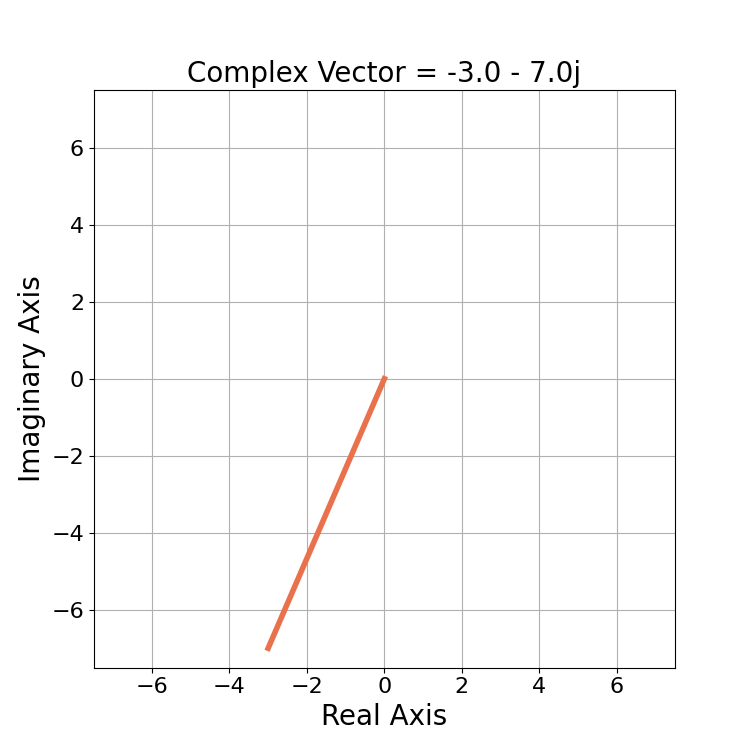The Fourier transform magnitude can be thought of as the length of the complex vector of X(e(j omega)) which is always a positive number.

The magnitudeis the distance from 0+0j to -3-7j which is

(9)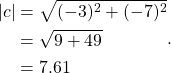#### Fourier Transform Magnitude

Using the representation in (2) for the complex number the magnitude can be written as

(10)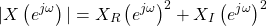which is always a positive number.

#### Conclusion

The magnitude of a complex number always has to be positive by definition, it can never be negative. The magnitude is conceptually the length of a complex vector which is written mathematically as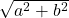.

More blogs on DSP:

Got a question? Drop it in the comments below or send me an email: matt@wavewalkerdsp.com

God, the Lord, is my strength; he makes my feet like the deer’s; he makes me tread on my high places. Habakkuk 3:19

### One Response

1.Chad M Spooner says:

I definitely agree that the magnitude of the Fourier transform, or any complex number, is non-negative. But students or newcomers to DSP might be a bit confused about this statement: “the square root of a positive number is always positive.” Because square roots of positive numbers can be negative: -2 is a valid square root of 4. I think the difference is in the definition of “magnitude” (or “modulus” or “absolute value”) which is defined as the positive square root.

When we use the square-root symbol, and there is no ‘-‘ or ‘+’ in front of it, ‘+’ is assumed.

Interested readers might look into metric spaces and inner products:

https://en.wikipedia.org/wiki/Magnitude_(mathematics)

https://en.wikipedia.org/wiki/Euclidean_space#Euclidean_norm

This website participates in the Amazon Associates program. As an Amazon Associate I earn from qualifying purchases.